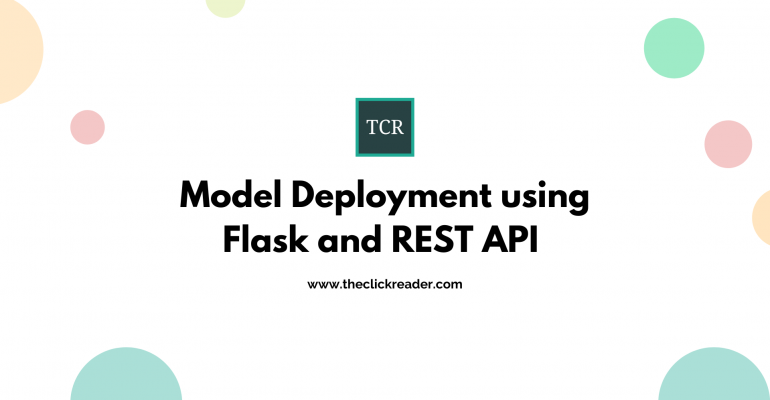Thanks to theidioms.com

Model Deployment using Flask and REST APIModel Deployment using Flask and REST API

It is not enough to build and train Machine Learning models. Your ML model is useless if it just sits in your PC after training. It would be more useful if we can deploy these models so that people can access them and make use of it, through which Machine learning can become a great tool for industries as well.

In this article We’ll see how to deploy a trained ML model using Python’s flask library. We’re assuming You already know how to make and train models.

Flask is a micro web framework written in Python. It is classified as a microframework because it does not require particular tools or libraries. It has no database abstraction layer, form validation, or any other components where pre-existing third-party libraries provide common functions. However, Flask supports extensions that can add application features as if they were implemented in Flask itself. Extensions exist for object-relational mappers, form validation, upload handling, various open authentication technologies and several common framework related tools.

Here are few reasons why we use Flask:

• It is easy to use
• Integrated unit testing support.
• Faster than Django.

ML Model

First, We’ll start with making the model and training it. For this example we’ll use breast-cancer database (https://raw.githubusercontent.com/apogiatzis/breast-cancer-azure-ml-notebook/master/breast-cancer-data.csv) for breast cancer detection model using scikit-learn module.

import pandas as pd

from sklearn.model_selection import train_test_split
from sklearn.ensemble import RandomForestClassifier
from sklearn import metrics

dataset_url = "https://raw.githubusercontent.com/apogiatzis/breast-cancer-azure-ml-notebook/master/breast-cancer-data.csv"

df['diagnosis']=df['diagnosis'].map({'M':1,'B':0}) // 1 to represent malignant

//preparing the data
train, test = train_test_split(df, test_size = 0.2)

//randomly selecting 5 features
features = ['texture_mean','perimeter_mean','smoothness_mean','compactness_mean','symmetry_mean']

train_X = train[features]
train_y = train.diagnosis

test_X = test[features]
test_y = test.diagnosis

model=RandomForestClassifier(n_estimators=100, n_jobs=-1)
model.fit(train_X,train_y)

prediction = model.predict(test_X)
metrics.accuracy_score(prediction,test_y)

Now, that our model is trained, we are ready to deploy it. But first We’ll save this model so that we don’t have to retrain model again and for this we’ll use pickle.

import pickle
filename = "model.sav"
pickle.dump(model, open(filename, 'wb'))

Now in a new file We will create a flask API app with 2 routes; one for home page and one for new data prediction through above model using POST HTTP method. So the basic structure of our app file will look like:

import pandas as pd
from sklearn.model_selection import train_test_split
from sklearn.ensemble import RandomForestClassifier
from sklearn import metrics
//render_template is used to render a HTML file
import re
import math

q = ""

@app.route("/")
return render_template('home.html', query="")

@app.route("/", methods=['POST'])
def cancerPrediction():
// code for model and new data prediction given in below steps
return render_template('home.html', output1=output, output2=output1, query1 = request.form['query1'], query2 = request.form['query2'],query3 = request.form['query3'],query4 = request.form['query4'],query5 = request.form['query5'])

app.run()

app.run()

Here, we’ll ask user for the values of 5 features so we create 5 input fields in HTML template and we’re throwing 2 outputs to whether it is cancerous or not and with how much probability. We’re using the following HTML as our frontend:

<html>
<script src="https://cdnjs.cloudflare.com/ajax/libs/popper.js/1.14.7/umd/popper.min.js"></script>
<script src="https://maxcdn.bootstrapcdn.com/bootstrap/4.3.1/js/bootstrap.min.js"></script>
<body>
<title>Breast Cancer Prediction</title>
<div class="container">
<div class="row">

<form action="http://localhost:5000" method="POST">
<div class="col-sm-9">
<div class="form-group  purple-border">
<label for="comment">texture_mean:</label>
<textarea class="form-control" rows="2" id="query1" name="query1" rows="2" cols="5" autofocus>{{query1}}</textarea>
</div>
<div class="form-group  purple-border">
<label for="comment">perimeter_mean:</label>
<textarea class="form-control" rows="2" id="query2" name="query2" rows="2" cols="5" autofocus>{{query2}}</textarea>
</div>
<div class="form-group  purple-border">
<label for="comment">smoothness_mean:</label>
<textarea class="form-control" rows="2" id="query3" name="query3" rows="2" cols="5" autofocus>{{query3}}</textarea>
</div>
<div class="form-group  purple-border">
<label for="comment">compactness_mean:</label>
<textarea class="form-control" rows="2" id="query4" name="query4" rows="2" cols="5" autofocus>{{query4}}</textarea>
</div>
<div class="form-group  purple-border">
<label for="comment">symmetry_mean:</label>
<textarea class="form-control" rows="2" id="query5" name="query5" rows="2" cols="5" autofocus>{{query5}}</textarea>
</div>
</div>
<div class="col-sm-3">
<button type="submit" class="btn btn-primary" name="submit">SUBMIT</button>
</div>
</form>
</div>

<div class="row">
<div class="col-sm-9">
<textarea class="form-control" rows="2" id="comment" name="query6" rows="2" cols="5" autofocus>{{output1}}</textarea>
<textarea class="form-control" rows="2" id="comment" name="query7" rows="2" cols="5" autofocus>{{output2}}</textarea>
</div>
</div>
</div>
</body>
</html>

So this is how the web application look like. We can add CSS or Bootstrap for a better look.

Now for the cancerPrediction() function we’ll use the same code we used for the model training, but this time to predict new values, we’ll take input as queries from the user. So put the following code inside cancerPrediction() function.

dataset_url = "https://raw.githubusercontent.com/apogiatzis/breast-cancer-azure-ml-notebook/master/breast-cancer-data.csv"
df.info()
//Input from form queries
inputQuery1 = request.form['query1']
inputQuery2 = request.form['query2']
inputQuery3 = request.form['query3']
inputQuery4 = request.form['query4']
inputQuery5 = request.form['query5']
df['diagnosis']=df['diagnosis'].map({'M':1,'B':0})

train, test = train_test_split(df, test_size = 0.2)

features = ['texture_mean','perimeter_mean','smoothness_mean','compactness_mean','symmetry_mean']

train_X = train[features]
train_y=train.diagnosis

test_X= test[features]
test_y =test.diagnosis

model=RandomForestClassifier(n_estimators=100, n_jobs=-1)
model.fit(train_X,train_y)

prediction=model.predict(test_X)
metrics.accuracy_score(prediction,test_y)

data = [[inputQuery1, inputQuery2, inputQuery3, inputQuery4, inputQuery5]]

# Create the pandas DataFrame
new_df = pd.DataFrame(data, columns = ['texture_mean', 'perimeter_mean', 'smoothness_mean', 'compactness_mean', 'symmetry_mean'])
single = model.predict(new_df)
probability = model.predict_proba(new_df)[:,1]
if single==1:
output = "The patient is diagnosed with Breast Cancer"
output1 = "Confidence: {}".format(probability*100)
else:
output = "The patient is not diagnosed with Breast Cancer"
output1 = ""

So after running the application by executing python app.py , we get these outputs on giving these values as input.

But right now we’re retraining every time the user is running the app, to avoid that we can use pickle.load() in our cancerPrediction() function and load the model which was saved previously as:

So, the final function looks like:

@app.route("/", methods=['POST'])
def cancerPrediction():
inputQuery1 = request.form['query1']
inputQuery2 = request.form['query2']
inputQuery3 = request.form['query3']
inputQuery4 = request.form['query4']
inputQuery5 = request.form['query5']

data = [[inputQuery1, inputQuery2, inputQuery3, inputQuery4, inputQuery5]]

# Create the pandas DataFrame
new_df = pd.DataFrame(data, columns = ['texture_mean', 'perimeter_mean', 'smoothness_mean', 'compactness_mean', 'symmetry_mean'])
single = model.predict(new_df)
probability = model.predict_proba(new_df)[:,1]

if single==1:
output = "The patient is diagnosed with Breast Cancer"
output1 = "Confidence: {}".format(probability*100)
else:
output = "The patient is not diagnosed with Breast Cancer"
output1 = ""

return render_template('home.html', output1=output, output2=output1, query1 = request.form['query1'], query2 = request.form['query2'],query3 = request.form['query3'],query4 = request.form['query4'],query5 = request.form['query5'])

So, this is how the Flask API deployment is done and the Machine learning Model is productized.